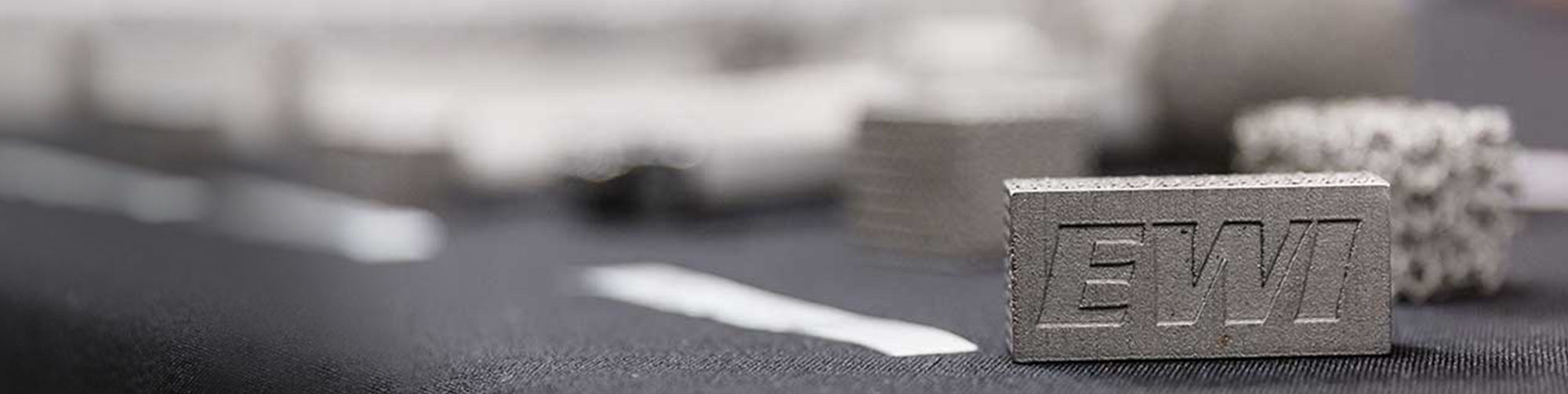# On Heating Mechanisms for Solid State Welding Processes

January 5, 2011

The majority of solid state welding processes use a combination of heat and deformation to accomplish an effective joint.  Heating is seen as both enabling to the necessary forging for joining, and in addition offers a diffusional component which enhances joint quality and reliability.  Solid state welding processes take advantage of a number of heat generation mechanisms.  While these mechanisms range from furnace and flame heating to percussive action, the dominant heat generation approaches used for solid state welding are either through resistive or deformation action.  Resistive heating is used in processes ranging from solid projection welding (RPW) to mash seam welding (RMSeW) to resistance butt or upset welding (UW).  Deformation related heat generation mechanisms are common to friction welding processes.   Heat generation through either of these mechanisms can be expressed in relatively simple terms.  Heat generation through resistance mechanisms is well understood and can be expressed as:

Where PΩ is power generation through resistance heating, I is the welding current, ρ is the workpiece resistivity, l is the heated length, and A is the conducting area.  Deformation related heating, however, is accomplished by the plastic working of the metal in the bond region.  In this case, power generation can be calculated through a combination of the local relative velocity of the workpieces and the shear strength of the material at temperature.  This can be expressed as:

Where Pτ is the power generation associated with the shearing action, τ is the shear stress at temperature, v is the surface velocity, and A is the area of the joint itself.  For linearly driven processes, this equation can be redefined as:

Where xm is the translation distance and f is the frequency.  Comparison of these heat generation mechanisms can be directly made by examining EWI’s new translationally assisted upset welding (TAUW) process.  This is a technology that combines both the resistance heating approaches of UW and the deformation heating approaches of translational friction welding (TFW).  A typical set of processing curves are shown in Figure 1 below.  By using the equations defined above, it can be defined that roughly 60% of the heat generated in this process is done through the current pulse, and 40% through the translational action as indicated in the plot.  The result is the joint shown in Figure 2.

Analyses such as these facilitate scaling of solid state welding processes, and are an enabler to developing newer and combined heat generation approaches in the future.Figure 2 - A Sample Weld Made using the Translationally Assisted Upset Process